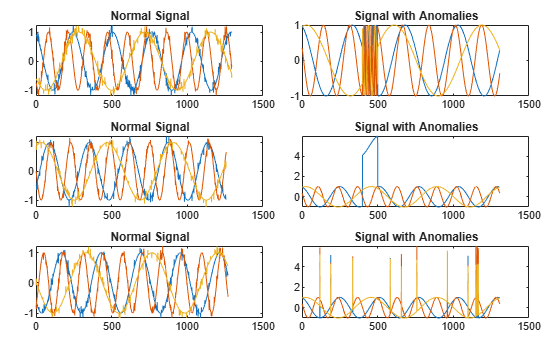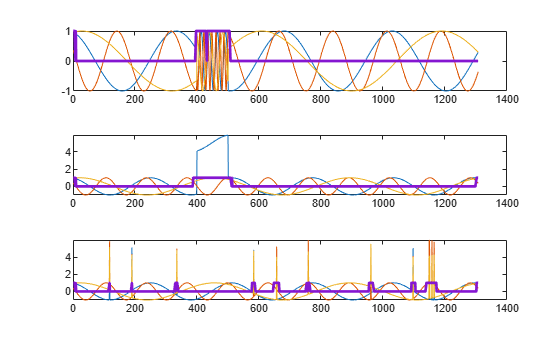# detect

Detect anomalies in signals

Since R2023a

## Syntax

``[lbls,loss] = detect(d,data)``

## Description

example

````[lbls,loss] = detect(d,data)` detects anomalies in signals stored in `data`.The function assigns a normal label to signal windows whose aggregated loss value is below the detection threshold, and an abnormal label to signal windows whose aggregated loss value is greater than or equal to the detection threshold.```

## Examples

collapse all

Load a convolutional anomaly detector trained with three-channel sinusoidal signals. Display the model, threshold, and window properties of the detector.

```load sineWaveAnomalyDetector D```
```D = deepSignalAnomalyDetectorCNN with properties: IsTrained: 1 NumChannels: 3 Model Information ModelType: 'conv' FilterSize: 8 NumFilters: 32 NumDownsampleLayers: 2 DownsampleFactor: 2 DropoutProbability: 0.2000 Threshold Information Threshold: 0.0510 ThresholdMethod: 'contaminationFraction' ThresholdParameter: 0.0100 Window Information WindowLength: 1 OverlapLength: 'auto' WindowLossAggregation: 'mean' ```

Load the file `sineWaveAnomalyData.mat`, which contains two sets of synthetic three-channel sinusoidal signals.

• `sineWaveNormal` contains the 10 sinusoids used to train the convolutional anomaly detector. Each signal has a series of small-amplitude impact-like imperfections but otherwise has stable amplitude and frequency.

• `sineWaveAbnormal` contains three signals of similar length and amplitude to the training data. One of the signals has an abrupt, finite-time change in frequency. Another signal has a finite-duration amplitude change in one of its channels. A third has random spikes in each channel.

Plot three normal signals and the three signals with anomalies.

```load sineWaveAnomalyData tiledlayout(3,2,TileSpacing="compact",Padding="compact") rnd = randperm(length(sineWaveNormal)); for kj = 1:3 nexttile plot(sineWaveNormal{rnd(kj)}) title("Normal Signal") nexttile plot(sineWaveAbnormal{kj}) title("Signal with Anomalies") end```Use the trained anomaly detector to detect the anomalies in the abnormal data.

`[lbls,loss] = detect(D,sineWaveAbnormal);`

The first output of `detect` is a categorical array that declares each sample of a signal as being anomalous or not.

```tiledlayout("vertical") for kj = 1:3 nexttile plot(sineWaveAbnormal{kj}) hold on plot(lbls{kj},LineWidth=2) end```## Input Arguments

collapse all

Anomaly detector, specified as a `deepSignalAnomalyDetectorCNN` or `deepSignalAnomalyDetectorLSTM` object. Use the `deepSignalAnomalyDetector` function to create `d`.

Signal data set, specified as one of these:

• Nc-column matrix — A single multichannel signal observation (M = 1), where Nc is equal to the value of the `NumChannels` property of the detector.

• M-element cell array — M multichannel signal observations, where each cell contains an Nc-column matrix.

• Timetable — A single multichannel signal observation, contained in a MATLAB® timetable. The timetable must contain increasing, uniformly-sampled, and finite values. The timetable can have:

• A single variable containing an Nc-column matrix, where each column corresponds to a signal channel.

• Nc variables, where each variable contains a vector that corresponds to a signal channel.

• Datastore — A `signalDatastore`, `audioDatastore` (Audio Toolbox), or `arrayDatastore` object. The detector uses the `readall` function to read all the signal observations contained in the datastore at once. You can also use a `CombinedDatastore` or `TransformedDatastore` object containing any of the supported datastores.

### Name-Value Arguments

Specify optional pairs of arguments as `Name1=Value1,...,NameN=ValueN`, where `Name` is the argument name and `Value` is the corresponding value. Name-value arguments must appear after other arguments, but the order of the pairs does not matter.

Example: `MiniBatchSize=64,ExecutionEnvironment="cpu"` instructs the function to use a mini-batch size of 64 and use the computer CPU to detect anomalies.

Option to expand window labels to each sample, specified as either `false` or `true`.

• If you specify `ExpandWindowLabels` as `false`, then `lbls` contains one label per window.

• If you specify `ExpandWindowLabels` as `true`, then `lbls` contains one label per sample.

Data Types: `logical`

Label priority for overlapped windows when `ExpandWindowLabels` is set to `true`, specified as `"anomaly"` or `"normal"`.

• If you specify `OverlapPriority` as `"anomaly"`, the function labels samples in an abnormal window overlapped with a normal window as abnormal.

• If you specify `OverlapPriority` as `"normal"`, the function labels samples in a normal window overlapped with an abnormal window as normal.

This argument applies only when `ExpandWindowLabels` is set to `true` and the window overlap length is greater than zero.

Data Types: `char` | `string`

Mini-batch size used by the network to compute reconstructed signals, specified as a positive integer scalar.

Data Types: `single` | `double` | `int8` | `int16` | `int32` | `int64` | `uint8` | `uint16` | `uint32` | `uint64`

Execution environment used by the network, specified as one of these:

• `"auto"` — If available, use the GPU. If the GPU is not available, use the CPU.

• `"gpu"` — Use the GPU.

• `"cpu"` — Use the CPU.

Data Types: `char` | `string`

## Output Arguments

collapse all

Labels, returned as a logical column vector, cell array, or timetable. The output format of `lbls` depends on the format of `data`.

• If `data` is an Nc-column matrix, then `lbls` is a logical column vector.

• If `data` is an M-element cell array, then `lbls` is a cell array containing M logical column vectors.

• If `data` is a timetable, then `lbls` is a timetable with a single variable containing a logical column vector.

• If `data` is a datastore, then `lbls` is a vector or cell array depending on the format of the data contained in the datastore.

A normal label has a value equal to `false`, and an abnormal label has a value equal to `true`.

Window loss, returned as a column vector, cell array, or timetable. The output format of `loss` depends on the format of `data`:

• If `data` is an Nc-column matrix, then `loss` is a single-precision column vector.

• If `data` is an M-element cell array, then `loss` is a cell array containing M single-precision column vectors.

• If `data` is a timetable, then `loss` is a timetable with a single variable containing a single-precision column vector.

• If `data` is a datastore, then `loss` is a vector or cell array depending on the format of the data contained in the datastore.

## Version History

Introduced in R2023a